CAT  >  Test Level 1: Percentages - 1

# Test Level 1: Percentages - 1

Test Description

## 10 Questions MCQ Test Level-wise Tests for CAT | Test Level 1: Percentages - 1

Test Level 1: Percentages - 1 for CAT 2023 is part of Level-wise Tests for CAT preparation. The Test Level 1: Percentages - 1 questions and answers have been prepared according to the CAT exam syllabus.The Test Level 1: Percentages - 1 MCQs are made for CAT 2023 Exam. Find important definitions, questions, notes, meanings, examples, exercises, MCQs and online tests for Test Level 1: Percentages - 1 below.
Solutions of Test Level 1: Percentages - 1 questions in English are available as part of our Level-wise Tests for CAT for CAT & Test Level 1: Percentages - 1 solutions in Hindi for Level-wise Tests for CAT course. Download more important topics, notes, lectures and mock test series for CAT Exam by signing up for free. Attempt Test Level 1: Percentages - 1 | 10 questions in 20 minutes | Mock test for CAT preparation | Free important questions MCQ to study Level-wise Tests for CAT for CAT Exam | Download free PDF with solutions
 1 Crore+ students have signed up on EduRev. Have you?
Test Level 1: Percentages - 1 - Question 1

### Raghu bought 4 dozens of oranges at Rs. 12 per dozen and 2 dozens of oranges at Rs. 16 per dozen. He sold them all and earned a profit of 20%. At what price per dozen did he sell the oranges?

Detailed Solution for Test Level 1: Percentages - 1 - Question 1

Total CP = Rs. (12 × 4 + 16 × 2) = Rs. (48 + 32) = Rs. 80
SP after profit = Rs. (1.2 × 80) = Rs. 96
SP per dozen = Rs. 96/6 = Rs. 16

Test Level 1: Percentages - 1 - Question 2

### If A is 1.5 times B, then B is what percent of A + B?

Detailed Solution for Test Level 1: Percentages - 1 - Question 2

According to the question,
A = 1.5 B
Now,
Required percentage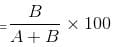Test Level 1: Percentages - 1 - Question 3

### A man buys a mobile phone at Rs. 5436 including 12.5% tax. How much money could he have saved by not paying the tax?

Detailed Solution for Test Level 1: Percentages - 1 - Question 3

Suppose, price of the mobile phone without tax is x.
Then, according to the question: x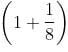= 5436
⇒ x = 5436 × 8/9 = 604 × 8 = 4832
∴ Price of the mobile phone without tax = 4832
∴ Amount of tax paid = 5436 - 4832 = Rs. 604
Alternative solution:
With tax of 12.5% = Rs. 5436
Since, 12.5% can be written as 1/8 part.
So, 1/(1 + 8) part of 5436 is the tax.
5436/9 = 604
Tax = Rs. 604

Test Level 1: Percentages - 1 - Question 4

B is 30% taller than A, and C is 30% shorter than B. By approximate how much percentage is A taller than C?

Detailed Solution for Test Level 1: Percentages - 1 - Question 4

If we assume A = 100 cm,
B will be = 100 × 1.3 = 130 cm.
Then, C will be = 130 × 0.7 = 91 cm.
(Since, C is 30% shorter than B, so 1 - 0.3 = 0.7)
Required percentage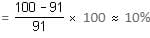Test Level 1: Percentages - 1 - Question 5

x is a positive integer. How many values can 'x' take such that x% of 250 is less than 60?

Detailed Solution for Test Level 1: Percentages - 1 - Question 5

x% of 250 should be less than 60.
x% of 250 < 60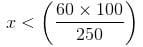x < 24
∴ x should be less than 24.
It can be any integer from 1 to 23.
So, it can take 23 values.

Test Level 1: Percentages - 1 - Question 6

In an election, there are two candidates A and B. Candidate A gets 40% of the total votes and loses the election by 9800 votes. Find the total number of votes.

Detailed Solution for Test Level 1: Percentages - 1 - Question 6

Let the total votes be x.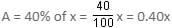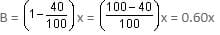Total votes by which Candidate B won = 0.6x - 0.4x = 9800
⇒ 0.2x = 9800
x = 9800/0.2 = 49,000

Test Level 1: Percentages - 1 - Question 7

The length of a cuboid is doubled, the breadth is tripled and the height is halved. Find the percentage increase in its volume.

Detailed Solution for Test Level 1: Percentages - 1 - Question 7

l1 = l; b1 = b; h1 = h
V1 = lbh
l2 = 2l; b2 = 3b; h2 = h/2
V2 = 3lbh
Increase in volume = 2lbh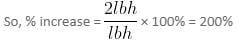Test Level 1: Percentages - 1 - Question 8

In a test, Ram got 40 marks and failed by 40 marks. If the pass percentage was 40%, then find the maximum marks.

Detailed Solution for Test Level 1: Percentages - 1 - Question 8

Let x be the maximum marks.
Passing marks = 40 + 40 = 80
80 = 40% of x (as given)
80 = 40/100 of x
x = 80/0.4 = 200

Test Level 1: Percentages - 1 - Question 9

At Infosys, 60% of the employees are males and the rest are females. Further, 20% of the males and 15% of the females are getting bonus. If the number of employees getting bonus is 81, then find the total number of employees who are not getting bonus.

Detailed Solution for Test Level 1: Percentages - 1 - Question 9

Let there be 100 employees at Infosys.
Number of male employees = 60
Number of female employees = 40
Total number of employees who are getting bonus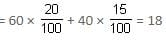If there are 100 employees, then number of employees getting bonus = 18
If 81 employees are getting bonus, then total number of employees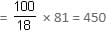So, total number of employees who are not getting bonus = 450 - 81 = 369

Test Level 1: Percentages - 1 - Question 10

It costs Rs. 5,000 to paint the total surface of a cube. But in summers, each side of the cube expands by 20%. What will be the cost of painting the cube in summers?

Detailed Solution for Test Level 1: Percentages - 1 - Question 10

The total surface area of cube = 6a2
Since each side expands by 20%, new side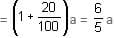∴ Total surface area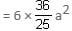So, the cost also increases by 36/25 times.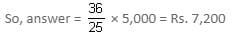## Level-wise Tests for CAT

5 docs|272 tests
 Use Code STAYHOME200 and get INR 200 additional OFF Use Coupon Code
Information about Test Level 1: Percentages - 1 Page
In this test you can find the Exam questions for Test Level 1: Percentages - 1 solved & explained in the simplest way possible. Besides giving Questions and answers for Test Level 1: Percentages - 1, EduRev gives you an ample number of Online tests for practice

## Level-wise Tests for CAT

5 docs|272 tests

### How to Prepare for CAT

Read our guide to prepare for CAT which is created by Toppers & the best Teachers